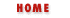# 6. Functions

Contents:
Defining and Invoking Functions
Functions as Data Types
The Function Object
Built-in Functions
Event Handlers

Functions are an important and complex part of the JavaScript language. This chapter examines functions from several points of view. First, functions are introduced from the syntactic standpoint, explaining how functions are defined and invoked. Second, it is shown that functions are data types in JavaScript, with examples of the useful programming techniques that are possible by treating functions as data. Finally, the Function object and its properties are discussed, which support a number of advanced techniques for manipulating functions and their arguments.

Functions in JavaScript are closely integrated with JavaScript objects, and there are features of functions that are not documented in this chapter. Chapter 7, Objects, explains the specialized uses of functions as methods, constructors, and event-handlers.

## 6.1 Defining and Invoking Functions

As we saw in Chapter 5, Statements, functions are defined with the function keyword, followed by:

• the name of the function

• a comma-separated list of argument names in parentheses

• the JavaScript statements that comprise the body of the function, contained within curly braces

Example 6-1 shows the definition of several functions. Although these functions are short and very simple, they all contain each of the elements listed above. Note that functions may be defined to expect varying numbers of arguments, and that they may or may not contain a return statement. The return statement was introduced in Chapter 5, Statements; it causes the function to stop executing and return the value of its expression (if any) to the caller. If a function does not contain a return statement, then it simply executes each statement in the function body and returns no value to the caller.

#### Example 6-1: Defining JavaScript Functions

```// A short-cut function, sometimes useful instead of document.write()
// This function has no return statement, so it returns no value.
function print(msg)
{
document.write(msg, "<BR>");
}
// A function that computes and returns the distance between two points.
function distance(x1, y1, x2, y2)
{
var dx = (x2 - x1);
var dy = (y2 - y1);
return Math.sqrt(dx*dx + dy*dy);
}
// A recursive function (one that calls itself) that computes factorials.
// Recall that x! is the product of x and all positive integers less than it.
function factorial(x)
{
if (x <= 1)
return 1;
else
return x * factorial(x-1);
}
```

Once a function has been defined, it may be invoked with the () operator, introduced in Chapter 4, Expressions and Operators. Recall that the parentheses appear after the name of the function, and that a comma-separated list of argument values (or expressions) appear within the parentheses. The functions defined in Example 6-1 could be invoked with code like the following:

```print("Hello, " + name);Summary of JavaScript Statements Book Index Functions as Data Types